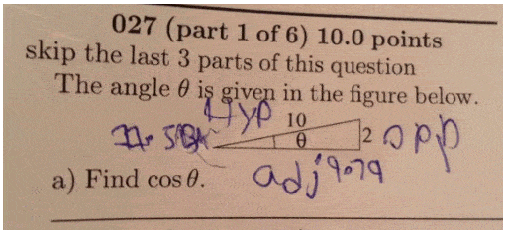# Need help with cosine problem?

[Note: Image orientation fixed, image trimmed to just relevant section - gneill]

## Homework Statement

hypotenuse is 10 opposite is 2 adjacent is unknown ( obviously needed for cosine)## Homework Equations

Sine=opposite/hypotenuse

## The Attempt at a Solution

#### Attachments

Last edited by a moderator:

gneill
Mentor
Hello edobbs0916, Welcome to Physics Forums.

Your post would be better placed in the Precalculus Mathematics Homework forum, so I have moved it there.

Also, as a hint, in a right angle triangle if you have two sides you can always calculate the third. So you need one more Relevant Equation...

RUber
Homework Helper
There are plenty of options here.
In the image, it seems like you have already found the length of the adjacent side (rounded improperly). So you could just go with what you know and check your arithmetic.
You could also use the relationship that ## \sin^2\theta + \cos^2\theta = 1## for any angle ##\theta##, so if you know sin, you can quickly know cos.